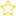See the live dashboard for CensusAtSchool 2023

This page (in progress) is aimed at helping teachers educate themselves about probability. Call back from time to time as it will continually be improved.

If you master the materials here you can be confident that you have enough content knowledge (in contrast to pedagogical knowledge) to teach probability up to year 13. The materials go a bit further than Year 13 but not much further.

Over view of probability in the NZ curriculum

If you want a very quick exposure to some probability ideas before starting on something more comprehensive, see these two videos from Dr Nic’s Basics of Probability playlist on her YouTube channel (contain adds)

Book chapter on probability (pdf) by Chris Wild & George Seber

This comprehensive resource is a pre-publication draft of the probability chapter for the book “Chance Encounters“. It goes a bit further than you need for Year 13 but it fills in a lot of the gaps and the motivations for what is done in the resources below. All of the answers to the exercises in the published chapter are here. Associated Powerpoint slides are here.

It does not explicitly contrast the notion of a true (but unknown) probability with probabilities from models (like equally-likely event models) and probabilities for data (obtained by doing something over and over again) as ways of obtaining approximations to a true probability.  See the paragraph on true probability below.
Additionally, it does its more complex calculations for conditional probabilities using tree diagrams instead of simpler methods based on 2-way tables.  Replacement pages that use 2-way tables are provided here. (The answers to the Exercises also use 2-way tables.)

Probability Lectures from the Stats 10x at Auckland

These Lectures are from the Stats 10x team at Auckland. They cover much of what you need and the ideas are very carefully explained by Matt Regan. You may prefer to view these videos before attacking the chapter above.

• Powerpoint Slides (used in the following videos)
• Accompanying Notes — the slides and videos assume the viewer is looking at these
• Gapped version (doc, pdf) for students, or use while viewing videos
• Filled in version(doc, pdf)
• Zip file for download — containing both the Powerpoint slides and the Word documents
• Screen capture videos of Matt Regan’s lectures (2012).
• Video 1  [56 mins mins] (Slides 1-47)
• Video 2  [15 mins] (Slides 51-70)
• Video 3  [26 mins] (Slides 71-153)
• Video 4  [52 mins] (Slides 154 – 179)
• Video on “Apply probability concepts in solving problems” by Marion Steele with associated resources

•
Understanding true probability, model estimates, and experimental estimates

Probability Resources

• Keywords: Experimental Probability, Probability
• (Last updated: 22/09/19. Added: 22/09/19)
• ## Rate this resource:(No ratings yet)